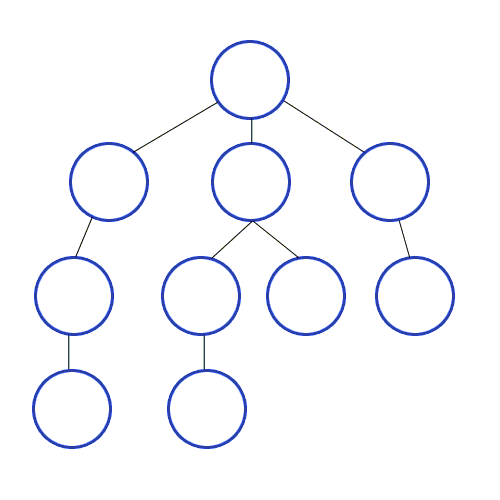### Archive

Posts Tagged ‘Goal node (computer science)’

## A* SearchImage via Wikipedia

A* uses a best-first search and finds the least-cost path from a given initial node to one goal node (out of one or more possible goals).

It uses a distance-plus-cost heuristic function (usually denoted f(x)) to determine the order in which the search visits nodes in the tree. The distance-plus-cost heuristic is a sum of two functions:

• The path-cost function, which is the cost from the starting node to the current node (usually denoted g(x))
• In addition, an admissible “heuristic estimate” of the distance to the goal (usually denoted h(x)).

## Depth First Search